# matlab二维数组的创建及其变换

1、MATLAB中，一般使用方括号（[]），逗号（，），分号（；）与空格来创建二维数组。空数组是一种非常特殊的数组，没有任何元素。创建一个空数组：只要把一个方括号复制给一个变量就可以了。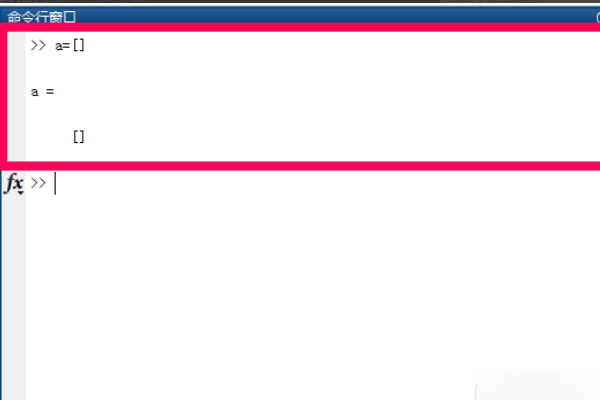2、在MATLAB中，创建二维数组可以使用空格+分号的形式。每一行的各个元素使用空格隔开，换行时使用分号隔开。需要注意的是，每一行的元素个数需要相同，不相同的情况下容易报错。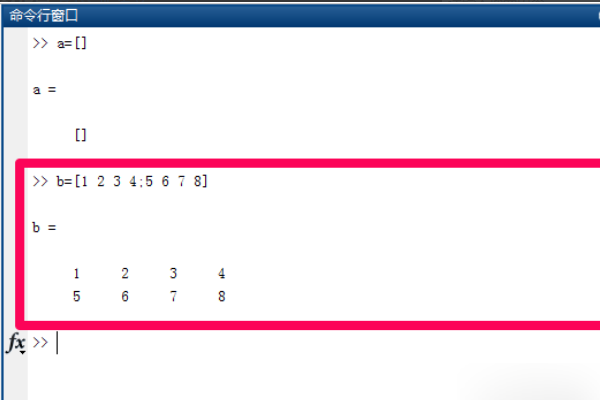3、在MATLAB中，也可以使用逗号+分号的形式创建数组。每一行的各个元素使用逗号隔开，换行时使用分号隔开。每一行的元素个数需要相同，如果每一行的元素个数不同，MATLAB会报错。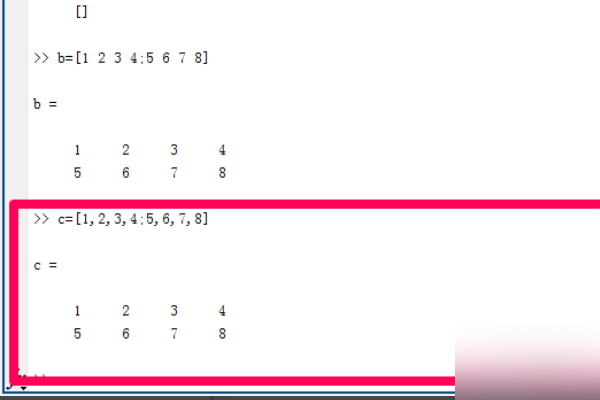4、在MATLAB中，可以使用冒号创建数组。A=a:b表示从实数a到实数b创建一个间隔为1的数组A。使用上述方式创造的数组A是一维数组，也可以被叫做行向量。数组A相邻两个元素的差值默认为1。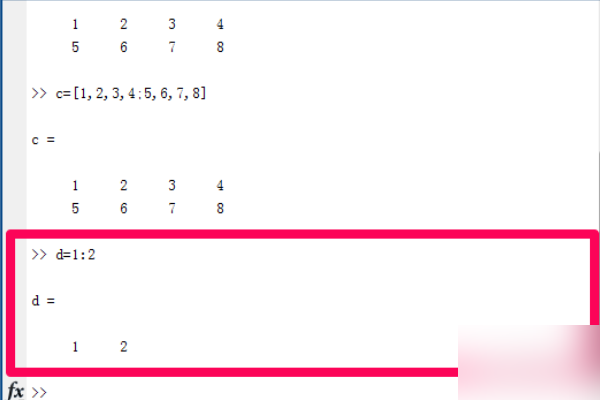5、也可以使用一种更加自由的方式，创建间距固定的递增或递减数组。A=a🅱️c表示从实数a到实数b以间隔为c递增或者递减，创造出数组A。使用这种方法创建数组时，数组A的第一个元素与最后一个元素分别是a和b，数组相邻两个元素之间的差值为c。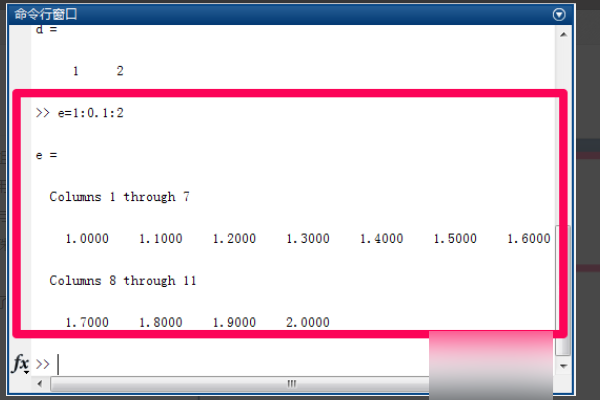A = [12 62 93 -8 22; 16 2 87 43 91; -4 17 -72 95 6]
A =
12 62 93 -8 22
16 2 87 43 91
-4 17 -72 95 6

A1=[1,2,3;4,5,6;7,8,9];A2=A1’;
A3=cat(2,A1,A2)
A3 =
1 2 3 1 4 7
4 5 6 2 5 8
7 8 9 3 6 9

1.矩阵的变维

（1）“：”变维

A=[1 2 3 4 5 6;6 7 8 9 0 1]
A =
1 2 3 4 5 6
6 7 8 9 0 1

B=ones(3,4)
B =
1 1 1 1
1 1 1 1
1 1 1 1

B(:)=A(😃
B =
1 7 4 0
6 3 9 6
2 8 5 1
（2）Reshape函数变维

B = reshape(A,m,n,p,…) %将矩阵A变维为m×n×p×…
B = reshape(A,[m n p…]) %同上
B = reshape(A,siz) %由siz决定变维的大小，元素个数与A中元素个数

a=[1:12];
b=reshape(a,2,6)
b =
1 3 5 7 9 11
2 4 6 8 10 12
2.矩阵的变向
（1）矩阵旋转

B = rot90 (A,k) %将矩阵A逆时针方向旋转(k×90°)，k可取正负整数。

A=[1 2 3;4 5 6;7 8 9]
A =
1 2 3
4 5 6
7 8 9

Y1=rot90(A),Y2=rot90(A,-1)
Y1 = %逆时针方向旋转
3 6 9
2 5 8
1 4 7
Y2 = %顺时针方向旋转
7 4 1
8 5 2
9 6 3
（2）矩阵的左右翻转

（3）矩阵的上下翻转

A=[1 2 3;4 5 6]
A =
1 2 3
4 5 6

B1=fliplr(A),B2=flipud(A)
B1 =
3 2 1
6 5 4
B2 =
4 5 6
1 2 3
（4）按指定维数翻转矩阵

A=[1 2 3;4 5 6]
A =
1 2 3
4 5 6

B1=flipdim(A,1),B2=flipdim(A,2)
B1 =
4 5 6
1 2 3
B2 =
3 2 1
6 5 4
（5）复制和平铺矩阵

B = repmat(A,[m n]) %与上面一致
B = repmat(A,[m n p…]) %B由m×n×p×…个A块平铺而成
repmat(A,m,n) %当A是一个数a时，该命令产生一个全由a组成的m×n矩阵。

A=[1 2;5 6]
A =
1 2
5 6

B=repmat(A,3,4)
B =
1 2 1 2 1 2 1 2
5 6 5 6 5 6 5 6
1 2 1 2 1 2 1 2
5 6 5 6 5 6 5 6
1 2 1 2 1 2 1 2
5 6 5 6 5 6 5 6
3．矩阵元素的数据变换

（1）按-∞方向取整

（2）按+∞方向取整

（3）四舍五入取整

（4）按离0近的方向取整

A=-1.5+4*rand(3)
A =
2.3005 0.4439 0.3259
-0.5754 2.0652 -1.4260
0.9274 1.5484 1.7856

B1=floor(A),B2=ceil(A),B3=round(A),B4=fix(A)
B1 =
2 0 0
-1 2 -2
0 1 1
B2 =
3 1 1
0 3 -1
1 2 2
B3 =
2 0 0
-1 2 -1
1 2 2
B4 =
2 0 0
0 2 -1
0 1 1
（5）矩阵的有理数形式

[n,d]=rat(A)
n =
444 95 131
-225 2059 -472
166 48 1491
d =
193 214 402
391 997 331
179 31 835
（6）矩阵元素的余数

©️2019 CSDN 皮肤主题: 大白 设计师: CSDN官方博客